# Price bundling

This article describes a pricing strategy used by sellers, typically in markets that suffer from imperfect competition, significant transaction costs or imperfect information.
View other pricing strategies

## Definition

Price bundling is a strategy whereby a seller bundles together many different goods/items being sold and offers the entire bundle at a single price.

There are two forms of price bundling -- pure bundling, where the seller does not offer buyers the option of buying the items separately, and mixed bundling, where the seller offers the items separately at higher individual prices. Mixed bundling is usually preferable to pure bundling, both because there are fewer legal regulations forbidding it, and because the reference price effect makes it appear even more attractive to buyers.

## Motivation behind price bundling

### Exploit different valuations by different buyers

#### Toy example

Suppose there are two buyers,$A$ and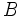$B$, and two products,$X$ and$Y$. Suppose buyer$A$ values product$X$ at$20$ units above the cost of production, and values$Y$ at$15$ units above the cost of production. Suppose buyer$B$ values$Y$ at$20$ units above the cost of production, and$X$ at$15$ units above the cost of production. Here is a simple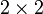$2 \times 2$ of the value the buyers place.

Value place on product$X$ above the cost of production (i.e., reservation price - cost of production) Value placed on product$Y$ above the cost of production Value placed on a bundle of$X$ and$Y$ above the cost of production = Sum of preceding two columns
Buyer$A$ 20 15 35
Buyer$B$ 15 20 35

The ideal thing for the seller would be to practice price discrimination: charge each buyer the maximum that buyer is willing to pay. However, this may be forbidden by law or otherwise difficult to implement.

Instead, the seller can pursue the following bundling strategy: charge slightly under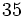$35$ units above production cost for the combination of$X$ and$Y$. Since both buyers value the combination at$35$ units above the cost of production, this deal appeals to both buyers. This allows the seller the obtain the entire social surplus as producer surplus. (It isn't true in general that bundling allows the seller to capture the entire social surplus -- that is a special feature of this situation because both buyers have similar reservation prices for the total bundle. However, bundling does allow the seller to capture more of the social surplus in many situations).

The seller can even make this a mixed bundling strategy: offer both$X$ and$Y$ individually for$20$ units above the cost of production, and offer the combination for slightly less than$35$ units above the cost of production.

#### Generalization

Consider the same two-buyer situation as above, but with more arbitrary numbers. Denote by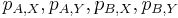$p_{A,X}, p_{A,Y}, p_{B,X}, p_{B,Y}$ the prices that buyers are willing to pay over and above the cost of production. Thus, for instance,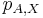$p_{A,X}$ is the maximum price that buyer$A$ is willing to pay for$X$ over and above the cost of production of$X$. We will assume that all four numbers are positive.

Here are the three basic cases:

Type of situation Seller's pricing strategy Seller's profit from buyer$A$ Seller's profit from buyer$B$ Seller's total profit
No price discrimination or price bundling Charge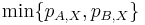$\min \{ p_{A,X}, p_{B,X} \}$ above cost of production for$X$ and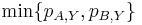$\min \{ p_{A,Y}, p_{B,Y} \}$ above cost of production for$Y$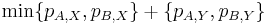$\min \{ p_{A,X}, p_{B,X} \} + \{ p_{A,Y}, p_{B,Y} \}$$\min \{ p_{A,X}, p_{B,X} \} + \{ p_{A,Y}, p_{B,Y} \}$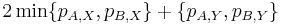$2\min \{ p_{A,X}, p_{B,X} \} + \{ p_{A,Y}, p_{B,Y} \}$
Pure price bundling Charge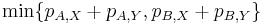$\min \{ p_{A,X} + p_{A,Y}, p_{B,X} + p_{B,Y} \}$ above cost of production for the bundle of$X$ and$Y$$\min \{ p_{A,X} + p_{A,Y}, p_{B,X} + p_{B,Y} \}$$\min \{ p_{A,X} + p_{A,Y}, p_{B,X} + p_{B,Y} \}$$2\min \{ p_{A,X} + p_{A,Y}, p_{B,X} + p_{B,Y} \}$
Perfect price discrimination Charge each buyer the maximum the buyer is willing to pay for each product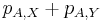$p_{A,X} + p_{A,Y}$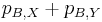$p_{B,X} + p_{B,Y}$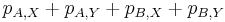$p_{A,X} + p_{A,Y} + p_{B,X} + p_{B,Y}$

With some basic algebra, we see that the pure price bundling strategy generates a profit intermediate between the strategy with no price discrimination or price bundling, and the strategy with perfect price discrimination. The following are the edge cases:

Conclusion Condition (verbal) Condition (algebraic)
Pure price bundling offers no advantage relative to no price discrimination or price bundling The higher-valuing buyer (among$A$ and$B$) for$X$ is the same as the higher-valuing buyer for$Y$, or one or both of$X$ and$Y$ is equally valued by both buyers.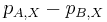$p_{A,X} - p_{B,X}$ and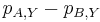$p_{A,Y} - p_{B,Y}$ have the same sign, or one or both of them equals zero.
Pure price bundling offers all the advantage of perfect price discrimination The bundle is equally valued by both buyers.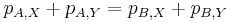$p_{A,X} + p_{A,Y} = p_{B,X} + p_{B,Y}$
All three cases coincide, i.e., no price discrimination, pure price bundling, and perfect price discrimination yield the same profit. The two buyers have identical willingness to pay.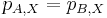$p_{A,X} = p_{B,X}$ and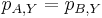$p_{A,Y} = p_{B,Y}$